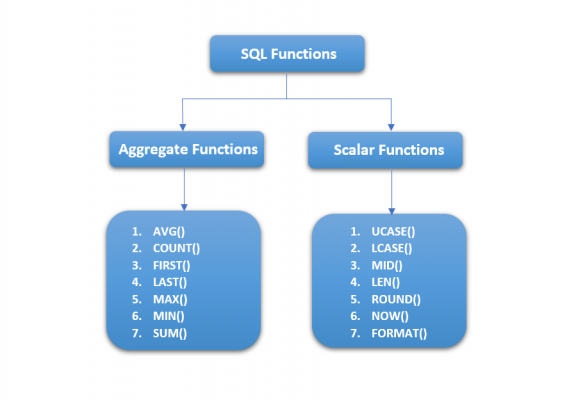# SQL Functions

SQL provides many built-in functions to perform calculations on data. Operations such as mathematical calculations, string manipulation like concatenation, substring are performed using these SQL Functions.

SQL Functions broadly can be divided into the following two categories.

1. Aggregate Functions
2. Scalar Functions### SQL Aggregate Functions

SQL Aggregate Functions return a single value after performing calculations on the multiple values. Following are the some of the most commonly used SQL Aggregate functions.

1. AVG() – It returns the average value.
2. COUNT() – It returns the number of rows.
3. FIRST() – It returns the first value.
4. LAST() – It returns the last value.
5. MAX() – It returns the largest value.
6. MIN() – It returns the smallest value.
7. SUM() – It returns the sum.

### SQL Scalar Functions

SQL Scalar Functions returns a single value based on the input provided. Here, the input also a single value. Following are the some of the most commonly used SQL Scalar Functions.

1. UCASE() – It converts a field to upper case.
2. LCASE() – It converts a field to lower case.
3. MID() – It extract characters from a text field.
4. LEN() – It returns the length of a text field.
5. ROUND() – It rounds a numeric field to the number of decimals specified.
6. NOW() – It returns the current system date and time.
7. FORMAT() – It formats how a field is to be displayed.